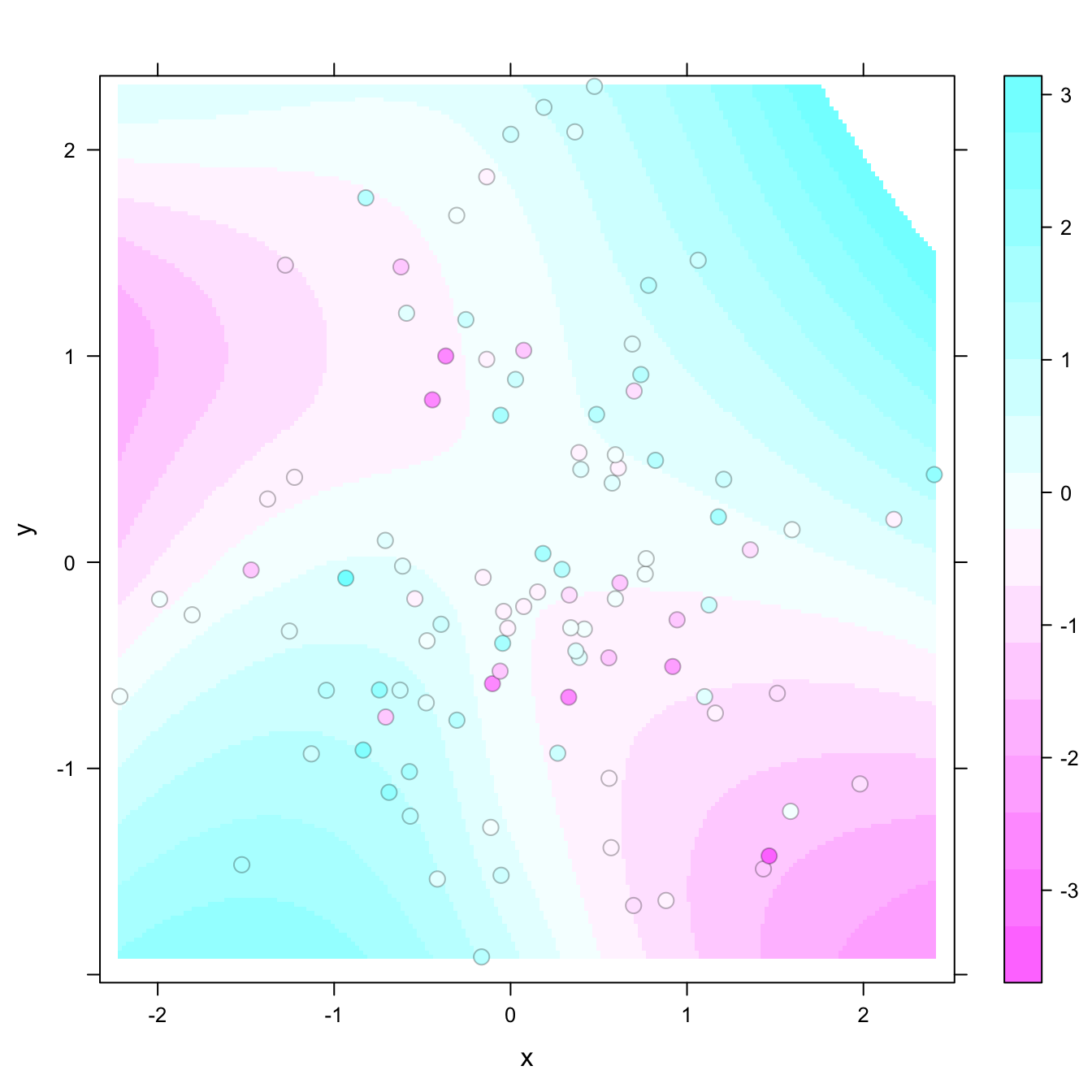# Heatmap with smoothing - LatticeExtra

The `latticeExtra` package allows to build a heatmap with smoothing. Here is how with reproducible code.

The levelplot function of lattice allows to deal with 3 numeric variables as shown in the heatmap section of the gallery.

Here, the idea is to plot data points as circles using `panel.levelplot.points` to get a scatterplot. Then, a layer is added using `panel.2dsmoother` to show the general distribution of the third numeric variable.``````# library
library(latticeExtra)

# create data
set.seed(1)
data <- data.frame(x = rnorm(100), y = rnorm(100))
data\$z <- with(data, x * y + rnorm(100, sd = 1))

# showing data points on the same color scale
levelplot(z ~ x * y, data,
panel = panel.levelplot.points, cex = 1.2
) +
layer_(panel.2dsmoother(..., n = 200))``````

Related chart types

## Contact

This document is a work by Yan Holtz. Any feedback is highly encouraged. You can fill an issue on Github, drop me a message on Twitter, or send an email pasting yan.holtz.data with gmail.com.